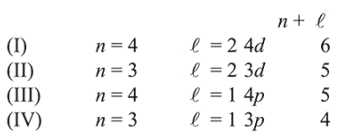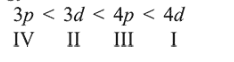# The quantum number of four electrons are given below :Question:

The quantum number of four electrons are given below :

I. $\quad n=4, l=2, m_{l}=-2, m_{s}=-1 / 2$

II. $n=3, l=2, m_{l}=1, m_{s}=+1 / 2$

III. $n=4, l=1, m_{l}=0, m_{s}=+1 / 2$

IV. $n=3, l=1, m_{l}=1, m_{\mathrm{s}}=-1 / 2$

The correct order of their increasing energies will be :

1. $\mathrm{IV}<\mathrm{III}<\mathrm{II}<\mathrm{I}$

2. $\mathrm{I}<\mathrm{II}<\mathrm{III}<\mathrm{IV}$

3. $\mathrm{IV}<\mathrm{II}<\mathrm{III}<\mathrm{I}$

4. $\mathrm{I}<\mathrm{III}<\mathrm{II}<\mathrm{IV}$

Correct Option: , 3

Solution:The energy of an atomic orbital increases with increasing $n+\ell$. For identical values of $n+\ell$, energy increases with increasing $\mathrm{n}$. Therefore the correct order of energy is: